# Box counting fractal dimension of volumetric data

Written by Paul Bourke
April-May 2014

Introduction

The box counting, or more precisely "cube counting" estimate for fractal dimension (FD) is also known as the Minkowski-Bouligand dimension or Kolmogorov dimension. The basic idea arises by considering the length, area, and volume of Euclidean objects such as a line, plane, and cube. In one dimension consider a curve and a ruler of length s. If one counts the number of rulers N(s) required to cover the curve as s decreases then the relationship will be N(s) proportional to 1/s1. Similarly in 2 dimensions if we count the number of squares of side length s required to cover a surface then the relationship will be N(s) proportional to 1/s2. And finally in 3 dimensions using a cube of side length s and counting how many such cubes are required to fill a volume the relationship will be N(s) proportional to 1/s3.

This is equivalent to our understanding of length, area, and volume. If we double the size of a one dimensional object the length increases by a factor of 21, if we double the size of a 2 dimensional object the area increases by a factor of 4 (22), and if we double the size of a 3 dimensional object the volume increases by a factor of 8 (23). The superscripts in the above correspond to the notion of dimensions 1, 2 and 3 respectively.

The above relationship can be generalised for fractal (fractional dimensional) objects by using the relationship N(s) proportional to 1/sD. If N(s) is computed across a range of s then there should be a linear relationship between log(N(s)) and log(1/s), the slope being the estimate of the fractal dimension. Absence of a linear relationship implies the object isn't fractal or it could be a multifractal.

Algorithm

Estimating the box counting FD of a 3D object involves counting the number of cubes N(s) of side length s required to cover the object. The FD is the slope of log(N(s)) vs log(1/s). One of the challenges is to decide how the data will be prepared and presented to the software. While image formats are well established to represent data for 2D FD estimates, the standards for volume data are less standardised. The choice used here is a simple raw format with a minimal header that contains the dimensions of the volume. The format chosen is the same used by the Drishti volume rendering software. This is a convenient because it is supported by the powerful Drishti data import software, allows simple checks to be performed on the volumes being analysed, and is quite easy to create using software one might write oneself. The following is a simplistic code snippet showing how the volume may be exported to the raw volume format chosen. In particular it defines the ordering of the x,y,z axes. The first byte defines the data type, 0 referring to an unsigned char. At the time of writing other datatypes are not supported. The volume dimensions are stored as integers (4 bytes). As such the header is 13 bytes followed by the raw volumetric data, namely nx*ny*nz bytes.

```   // The volume has dimensions nx,ny,nz
// The volume is of type unsigned char, for example, unsigned char ***vol
fputc(0,fraw);
fwrite(&nz,sizeof(int),1,fraw);
fwrite(&ny,sizeof(int),1,fraw);
fwrite(&nx,sizeof(int),1,fraw);
for (k=0;k<nz;k++) {
for (j=0;j<ny;j++) {
for (i=0;i<nx;i++) {
fputc(vol[i][j][k],fraw);
}
}
}
```

The algorithm is quite straightforward: over a range of offsets (see later) and over a range of cube sizes s, compute the number of cubes which contain a voxel within the object under study. One immediately realises that there needs to be a definition of what voxel values constitute the object, this is also known as thresholding and sometimes as segmentation. The key point is that box counting does not apply to a volume with continuous values but rather only to a binary volume. This thresholding can be performed external to this box counting software or by using the -level or -range command line options, see software usage below.

The algorithm may be written in pseudo-code as follows:

```   for all offsets
for all box sizes s
N(s) = 0
for all box positions
for all voxels inside the current box
if the voxel is part of the object
N(s) = N(s) + 1
stop searching voxels in the current box
end
end
end
end
end
```
Case study 1: Menger sponge

Before applying any fractal dimension estimator to data of unknown FD it is important to test the algorithm against fractals of known dimension. In the case of the 3D Menger sponge illustrated below the FD is given by log(20) / log(3) = 2.7268, this happens to be both the FD of the volume as well as the surface.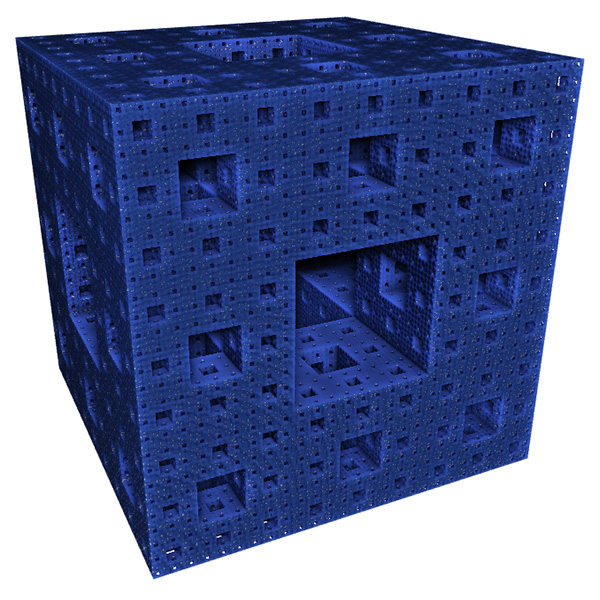The raw results reported by the software are shown in the table below. The first column is the box size s. The second column is the number of boxes of that size required to cover the object N(s). The third and fourth columns are log(1/s) and log(N(s)) respectively, the slope of these is the estimate of the box counting FD.

```  s      N(s)    log(1/s)    log(N(s))
---- ---------- ----------  -----------
2   14348848   -0.69314     16.4792
3    3200000   -1.09861     14.9787
4    2432160   -1.38629     14.7043
6     723680   -1.79176     13.4921
9     160000   -2.19722     11.9829
13     104736   -2.56495     11.5592
19      37044   -2.94444     10.5199
28      13635   -3.33220      9.5204
42       4400   -3.73767      8.3893
63       1458   -4.14313      7.2848
94        432   -4.54329      6.0684
141        200   -4.94876      5.2983
211         64   -5.35186      4.1588
```

The graph of the raw data above is shown below and the measured slope is 2.62. For these known cases one doesn't expect a perfect match to theoretical because the image is only an approximation to the real fractal form.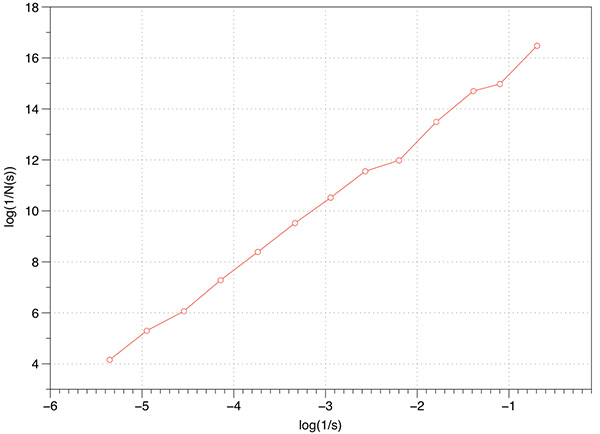In the above estimation a maximum of 1000 offsets were used to find the minimum coverage. Note that for box sizes 3 and 9 there is a round number of N which is due to the Menger sponge being aligned to voxels in it's generation. Just using these two data points the FD estimate would be the expected value.

(log(3200000) - log(160000)) / (log(1/3) - log(1/9)) = 2.7268

However, as it happens these two points are an artefact of the way the sponge was created, as such they actually serve to bias the result lower than the expected value.

Case Study 2: Cantor dust

Another 3D fractal of known FD is Cantor dust, this is formed in 1D by starting with a line segment and removing the middle 1/3 segment and iteratively for all subsequent line segments.This can readily be extended into 2D and 3D. The FD for the 3D case is log(8) / log(3) = 1.8928.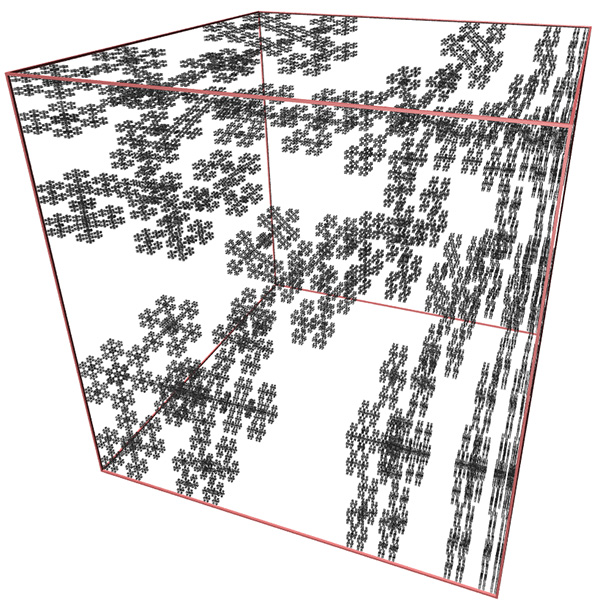The graph of log(N(s)) vs log(1/s) is shown below, a maximum of 1000 offsets were tested. The measured slope is 1.88 which is extremely good agreement with the expected value.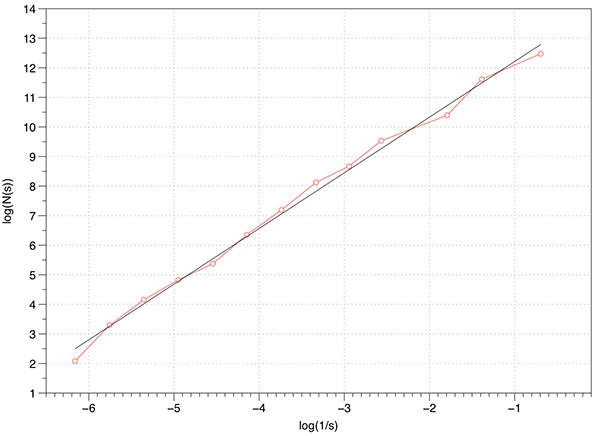Accurate estimates of the FD require one finds the minimum number of boxes that cover the object. Few algorithms seem to consider this, perhaps because it greatly increases the processing time required. In the implementation here, and since the data is based upon regular volumetric data, the origin for the 3D tile of boxes is randomly offset in the three dimensions. That is, for a particular box size many possible coverings are considered and the minimum is recorded for the subsequent slope estimation. A simple example is shown below in 2 dimensions, the origin for the boxes on the left gives N(s) = 4 whereas the minimum box count required to cover the object is N(s) = 2.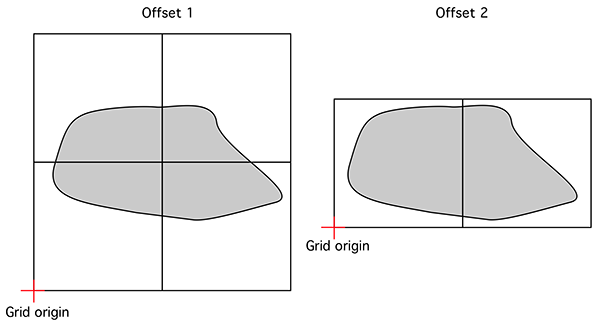The effect on the FD estimate by using offsets is explored below using the cantor dust example. As the box size increases there is more likelihood that lower box coverings will be found so the effect will be to generally raise the FD estimate. The small box sizes are less likely to change with higher offset counts. Put another way, without considering offsets any estimate of FD will be biased towards lower values. Low number of offsets additionally results in more variation of the slope and thus less reliable slope estimates.

An even more rigorous implementation to find the minimal coverage would include considering a range of angular orientations for the coordinate system. This not only adds another three degrees of freedom and the corresponding computation time, but also raises some questions around the fact that sample boxes no longer align in a discrete way with the volumetric data.Non-fractal tests

The three tests conducted below were for a line, plane, and a solid box. Of course the dimension of each of these is known, namely 1, 2, and 3 respectively. As shown below the estimates from the software were as close as can be expected for a limited sample (volume) size.

Line segment: FD estimate of 0.98.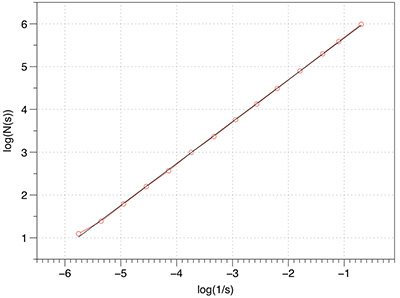Plane: FD estimate of 1.96.Box: FD estimate of 2.95.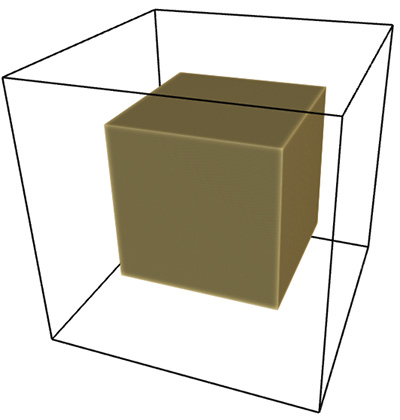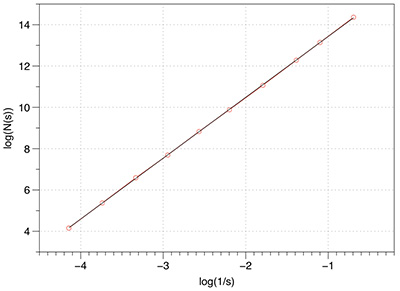Effect of volume resolution

As one might expect, the higher the volume resolution the better the FD estimate will be. The following graphs are for 1000 offsets for a cube object ranging from 20x20x20 to 640x640x640. As expected the variation of the slope reduces as the resolution increases.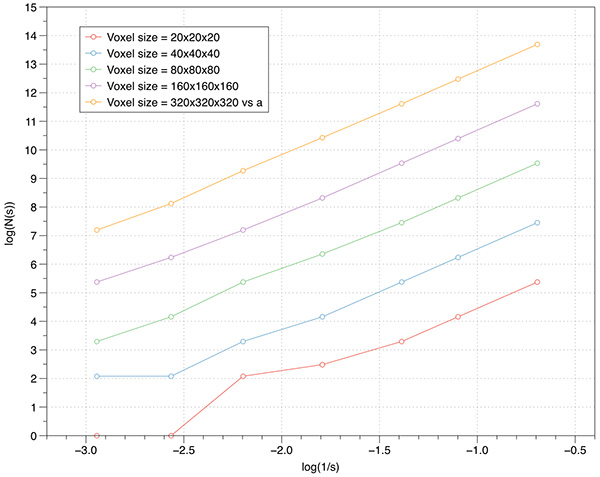Levels

Most volumetric data is of course not binary, many are continuous functions arising from CT or MRI scans. While volume processing software allows one to segment and otherwise threshold data, some of that is supported within the box counting software itself. One option is to set the level at which a voxel is decreed to be solid or not. By default since volume voxels are single bytes then the values range from 0 to 255, the threshold is taken to be 128. So one may run simple scripts that scan across a range of threshold levels computing the FD for each level.An example of this is demonstrated for the volume above. The application of this is to scan the volume for threshold ranges of interest from a FD perspective.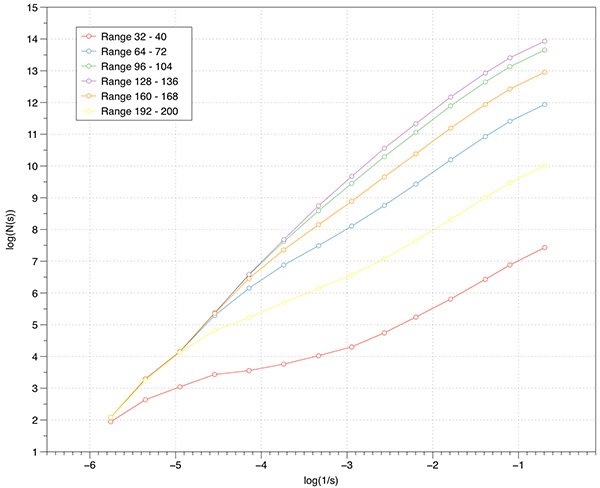Case study 3

Further tests can be performed using 2D fractals with known FD, for example the Menger carpet. The theoretical FD is log(8) / log(3) = 1.8928. An extruded Menger carpet would have a FD of 1 + log(8) / log(3) = 2.8928. The measured slope from the Menger sponge and extruded Menger sponge is 1.8 and 2.78 respectively.2D Menger carpet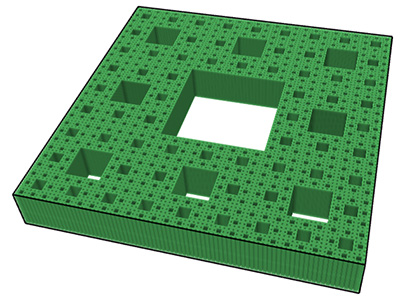2D Menger carpet extruded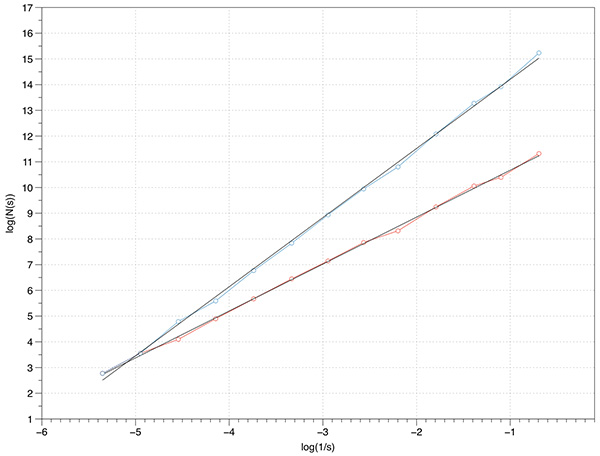Case study 4

Theoretical FD for the 2D case is log(8) / log(2) = 1.58, measured FD is 1.54. The theoretical FD for the extruded case is 2.58, measured is 2.5.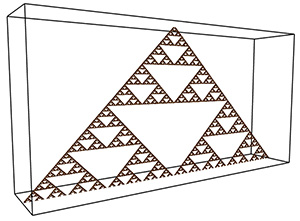```Usage: volboxcount [options] volfilename
Options:
-bmin n       minimal box size (default: 2)
-bmax n       maximum box size (default: largest dimension)
-bfact n      box size factor (default: 1.5)
-level n      voxel level above which defines the object (default: 128)
-range n1 n2  voxel range defining the object (default: 128 255)
-mset n       Maximum number of offsets used (default: 10)
```
Notes:

• -bmin is the lowest, initial box size. There is no point choosing a value less than 2 since such a choice would merely` result in counting the number of voxels contained in the object. Low box sizes tend to be counting the volume of the object and thus tend towards a bias of 3.

• -bmax is the largest box size considered. As the box size exceeds the dimensions of the volume the count will tend to 1 and log(N(s)) to zero.

• -bfact is the multiplicative increase in box size. So if the first box has side length s, and if the default factor of 1.5 is used then the next box size will be 1.5s, the next 1.52s, then 1.53s, and so on. This multiplicative increase assures regular steps on the log(N(s)) vs log(1/s) graph.

• -level sets a level below which a voxel is defined not be part of the object.• -range sets a lower and upper level, voxels between those levels belong to the object. Care should be taken when using anything other than a very narrow range as the FD will be biased towards 3 since one may have essentially a 3D volume. Note that -level and -range are exclusive and that "-level X" is shorthand for "-range X 255".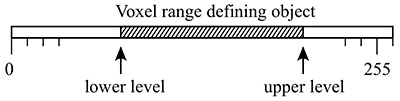Worked example

In what follows is a complete worked example showing all aspects of the software and analysis. The dataset being used can be downloaded from here: foam.raw. Rendered with Drishti and a transfer function centered on the value of 16 is illustrated below.The command line is as follows

```volboxcount -bmin 4 -bmax 50 -range 10 20 10 -mset 1000 foam.raw
```

The standard output is shown below, note that for a particular box size the multiple box counts occur when a lower number is found during the offset search.

```Computing box size:    4, Offset:    0 /   64, Minimum box count: 66316
Computing box size:    4, Offset:    1 /   64, Minimum box count: 65948
Computing box size:    4, Offset:    3 /   64, Minimum box count: 65520
Computing box size:    4, Offset:    6 /   64, Minimum box count: 65199
Computing box size:    4, Offset:   17 /   64, Minimum box count: 64923
Computing box size:    4, Offset:   63 /   64, Minimum box count: 64468
Computing box size:    6, Offset:    0 /  216, Minimum box count: 27134
Computing box size:    6, Offset:    1 /  216, Minimum box count: 27010
Computing box size:    6, Offset:    2 /  216, Minimum box count: 25829
Computing box size:    6, Offset:   37 /  216, Minimum box count: 25779
Computing box size:    6, Offset:   74 /  216, Minimum box count: 25678
Computing box size:    6, Offset:  197 /  216, Minimum box count: 25613
Computing box size:    9, Offset:    0 /  729, Minimum box count: 8657
Computing box size:    9, Offset:    4 /  729, Minimum box count: 8589
Computing box size:    9, Offset:    6 /  729, Minimum box count: 8248
Computing box size:    9, Offset:   71 /  729, Minimum box count: 7871
Computing box size:   13, Offset:    0 / 1000, Minimum box count: 3354
Computing box size:   13, Offset:    1 / 1000, Minimum box count: 3349
Computing box size:   13, Offset:    2 / 1000, Minimum box count: 3345
Computing box size:   13, Offset:    3 / 1000, Minimum box count: 3343
Computing box size:   13, Offset:    5 / 1000, Minimum box count: 3132
Computing box size:   13, Offset:    6 / 1000, Minimum box count: 3131
Computing box size:   13, Offset:    8 / 1000, Minimum box count: 3127
Computing box size:   13, Offset:   11 / 1000, Minimum box count: 2924
Computing box size:   13, Offset:   20 / 1000, Minimum box count: 2921
Computing box size:   13, Offset:   33 / 1000, Minimum box count: 2920
Computing box size:   13, Offset:  243 / 1000, Minimum box count: 2735
Computing box size:   13, Offset:  352 / 1000, Minimum box count: 2733
Computing box size:   13, Offset:  623 / 1000, Minimum box count: 2732
Computing box size:   13, Offset:  799 / 1000, Minimum box count: 2731
Computing box size:   19, Offset:    0 / 1000, Minimum box count: 1208
Computing box size:   19, Offset:    2 / 1000, Minimum box count: 1100
Computing box size:   19, Offset:    3 / 1000, Minimum box count: 1098
Computing box size:   19, Offset:   10 / 1000, Minimum box count: 1000
Computing box size:   19, Offset:   14 / 1000, Minimum box count: 999
Computing box size:   19, Offset:  348 / 1000, Minimum box count: 998
Computing box size:   28, Offset:    0 / 1000, Minimum box count: 447
Computing box size:   28, Offset:    2 / 1000, Minimum box count: 392
Computing box size:   28, Offset:    3 / 1000, Minimum box count: 343
Computing box size:   42, Offset:    0 / 1000, Minimum box count: 125
```

The raw data is as follows:

```  s      N(s)    log(1/s)    log(N(s))
---- ---------- ----------  -----------
4     64468    -1.38629     11.0739
6     25613    -1.79176     10.1509
9      7871    -2.19722     8.97094
13      2731    -2.56495     7.91242
19       998    -2.94444     6.90575
28       343    -3.33220     5.83773
42       125    -3.73767     4.82831
```

Graphed data shown below, FD estimate is 2.7.References

• Ankerst, M., Kastenmuller, G., Kriegel, H.P., Seidl, T.: 3D shape histograms for similarity search and classification in spatial databases. Guting, R.H., Papadias, D., Lochovsky, F.H. (eds.) SSD 1999. LNCS, vol. 1651, pp. 207-226. Springer, Heidelberg (1999)

• Backes, A.R., Casanova, D., Bruno, O.M.: Plant leaf identification based on volumetric fractal dimension. IJPRAI 23(6), 1145-1160 (2009)

• Backes, A.R., de Sa Jr., J.J.M., Kolb, R.M., Bruno, O.M.: Plant species identification using multi-scale fractal dimension applied to images of adaxial surface epidermis. Jiang, X., Petkov, N. (eds.) CAIP 2009. LNCS, vol. 5702, pp. 680-688. Springer, Heidelberg (2009)

• Jacques Soddell and Robert Seviour, Using Box Counting Techniques for Measuring Shape of Colonies of Filamentous Micro-organisms. Complexity International (1995) 2

• J. Ruiz de Miras, J. Navas, P. Villoslada, F.J. Esteban. UJA-3DFD:A program to compute the 3D fractal dimension from MRI data. Computer Methods and Programs in Biomedicine Volume 104, Issue 3 , Pages 452-460, December 2011

• M. Nezadal, O. Zmeskal, M. Buchnicek, The Box-Counting: Critical Study. Technical University of Brno, Faculty of Chemistry, Institute of Physical and Applied Chemistry, Purkynova 118, 612 00 Brno, Czech Republic

• J. W. Dietrich, A. Tesche, C. R. Pickardt and U. Mitzdorf. Fractal Properties of the Thyrotropic Feedback Control Implications of a Non-linear Model Compared with Empirical Data. Cybernetics and Systems, 2002